Shopping Cart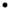Download Website
 You are here: Filter ASIC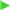Project Spec

 Filter ASICMain PageProject SpecCMOS Layout

# Original Project Specification

The original document written by Richard Schreier is available in Postscript format. This web page is more or less similar to the original specification distributed in January 1993.

## Important Information

### Timetable: TIGHT!

• The layout is due Monday March 1 at 9:30am.
• The project report is due March 5 at 5:00pm.
• The assignments during the term are geared toward helping you to complete the project on time. It is in your best interest to keep pace with them.

## General Information:

• Chip design will be done in pairs.
• For each design, MOSIS will give us 4 parts, but I will keep at least 1 from each group. The rest are yours.
• The chip is 2.2x2.2mm (a MOSIS TinyChip) and will be in a 28-pin DIP.
• The process is a 2.0m P-well, double-poly process.
• Vineet will provide the basic pad frame.
• You can (and should) use cells from the CMOSN library whenever possible.

### Project Contents:

• Your IC will contain a ring-oscillator with divide-by-32 circuitry, an RS latch (which you can use to generate a glitch-free clock during manual testing) and a decimator for a multi-bit delta-sigma modulator. Design of the decimator will constitute the major portion of the project. Detailed specifications for the decimator are given in the remainder of this handout. You should be aware that the specifications will likely change as you discover how difficult it is to squeeze all the customer's features into one TinyChip.

### Project Evaluation:

• Vineet will check the layout for DRC errors. There should be none. The bulk of the marks will be based on the report. I expect to see
1. A description of the function and I/O timing of your chip.
2. A plot of your chip and of the major cells.
3. Schematics of the cells used, annotated with transistor sizes.
4. Simulations and calculations. (Estimated ring-oscillator oscillation frequency, maximum clock speed, clock-buffer calculations etc.)
5. A description of what you did with your left-over area (if anything) and relevant calculations.

### Free Space:

• If there is free space, you may put anything you like in it. The fact that this is a double-poly process implies that you can implement analog functions using switched-capacitor circuits.

### Ring Oscillator: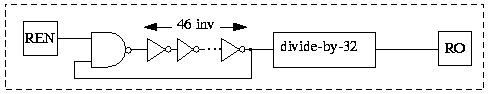Figure 1: When REN is high, the ring oscillator should oscillate, and its divided-down output should be available at RO.

### RS Latch: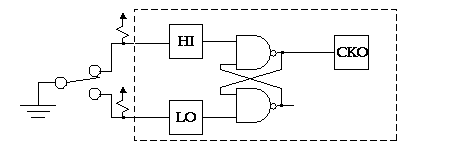Figure 2:An external toggle switch can be used to generate a glitch-free clock signal at CKO. This can be helpful during manual testing.

### Decimator: General Information

The main function of the chip is to act as a decimator for a multi-bit delta-sigma modulator. Don't worry if you don't understand the purpose of this block; you only need to understand its operation well enough to build it.

Figure 3 shows the block diagram of the decimator using digital signal processing (DSP) notation. If you don't know what represents, don't panic; you can view the formulae in the boxes simply as names. What you should observe from FigureĀ3 is that the incoming data, x, is passed through four identical blocks, then sub-sampled and passed through another four identical blocks to produce the output y. An alternative specification for the decimator is given in Figure 4, which specifies the operations of the decimator are with a C program.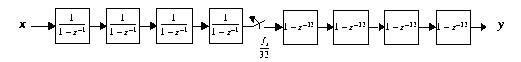Figure 3:The block diagram of the decimator in DSP notation.

Note: This C code is available plain or in ZIP format.

```/*
* decimate.c
* Simulate the mathematical operations carried out
* by a sinc^4 decimator with a decimation factor of 32.
*
* The decimator uses modulo 2^24 arithmetic to do
* its calculations.
*
* R. Schreier of OSU, 1993.
*/

#define R 32
#define N 24

static int Modulus = 1<<N;
static int Signbit = 1<<(N-1);

int mod( int x ){
}

main(){
/* Declarations. */
int a1=0, a2=0, a3=0, a4=0, s1=0, s2=0, s3=0, s4=0;
int t, x, y1, y2, y3, y4;

/*
printf("Signbit = %.8x\n", Signbit );
*/

/* Get the input and go through the equations. */
for(t=0; scanf("%d",&x) > 0; ){
a1 = mod( a1 + x );
a2 = mod( a2 + a1 );
a3 = mod( a3 + a2 );
a4 = mod( a4 + a3 );
if( ++t == R ){
t = 0;
y1 = mod( a4 - s1 );
s1 = a4;
y2 = mod( y1 - s2 );
s2 = y1;
y3 = mod( y2 - s3 );
s3 = y2;
y4 = mod( y3 - s4 );
s4 = y3;
if( y4 & Signbit )
printf( "%d\n", y4 - Modulus );
else
printf( "%d\n", y4 );
}
}
exit(0);
}
```
Figure 4:A C program which simulates the operation of the decimator. Source is available in ~schreier/ece517/decimate.c and the executable is decimate, in the same directory.

As your customer, I want the chip to accept data at a frequency of at least 10ĀMHz. For an input data width of 4 bits, I have determined that the internal data path needs to be 24 bits wide. If you can increase the internal word width, then the input data width can be increased and I will be happier. Six pins have therefore been allocated for the input data, even though I expect that you will only be able to accommodate four (or fewer). See Table 1 for a list of the pins and their functions.

Note that pins 12-18 have not been assigned. I expect that you will want to omit them, so as to give yourself more space on the IC for gates. See FigureĀ5 for the pad layout that was used last year. If you can cram all the functions I want into 1700l X 1700l, then you can add pads to give yourself more free pins. At the moment, only pin 26 is free, and I have specified it as an output. Vineet will issue more details on the pad frame when he finishes laying it out.

The output of the decimator is a 24-bit word, which will be output serially through the Y pin, with the LSB coming out first. To demarcate words, FS (frame sync) will be high when the LSB appears and low otherwise. The OK output is to be asserted at the end of the automatic self-test, which is initiated by TM (test mode) going high. Transitions on TM should reset the IC.

 Number Type Name Block Number Type Name Block 1 Input REN Ring Osc. 15 2 Input X0(lsb) Decimator 16 3 Input X1 Decimator 17 4 Input X2 Decimator 18 5 Input X3 Decimator 19 Output CKO RS Latch 6 Input X4 Decimator 20 Input HI RS Latch 7 Input X5 Decimator 21 Input LO RS Latch 8 VDD VDD 22 VDD VDD 9 VSS VSS 23 VSS VSS 10 Input CK Decimator 24 Output Y Decimator 11 Input TM Testing 25 Output FS Decimator 12 26 Output Spare Spare 13 27 Output OK Testing 14 28 Output RO Ring Osc.
Table 1: Pin Data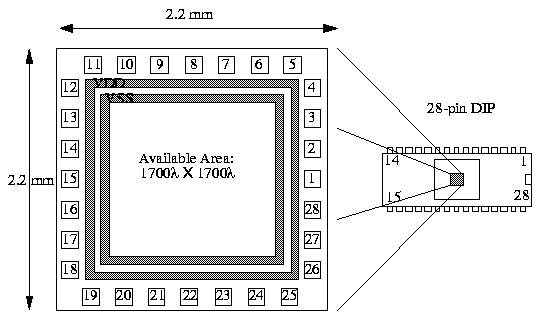Figure 5:The placement of a TinyChip inside a 28 pin DIP. Pins 12-18 may be omitted to increase the area available for gates.

### Decimator: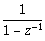block

This block, which I shall refer to as the summer block, produces as its output the sum of all previous inputs. It works by keeping a running total of its input and adding successive inputs to the running total. See FigureĀ6 for a digital-logic representation of the required function. The variables a1, a2, a3 and a4 in the C program represent the running totals of the four integrator blocks. Note that for correct operation of this block, the adder must "roll-over" when overflows occur. This is modelled in the C program by a modulo 224 operation on all variables. This operation guarantees that the integers in the C program contain at most 24 bits.Figure 6: The logic required for the summer block.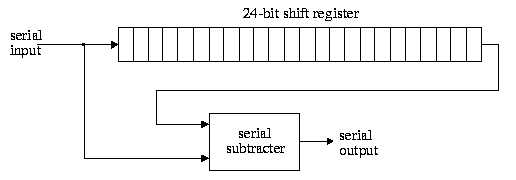Figure 7:Logic implementation of the subtracter block.

### Decimator: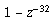block

The first block you will design (for Assignment 2) is the, or subtracter, block. This block produces as its output the difference between its two most recent inputs. Since the sample rate for the subtracters is of the sample rate for the summers, you can save hardware by making this block operate serially. FigureĀ7 gives a hint as to how you might implement the subtracter. It is up to you to determine the contents of the block labelled "serial subtracter".

In the C program, the variables s1, s2, s3 and s4 contain the values in the subtracter register and the variables y1, y2, y3 and y4 are the four subtracter outputs. Your design must operate in a manner that is consistent with the operation specified in the C program. (You should use the program to generate test data.) Be careful to reset the state of the serial subtracter before each cycle.

### Decimator: Test mode

To make testing of your IC trivial, I would like it to contain built-in self-test (BIST) circuitry. We will cover this later in the course. The idea in a nutshell is to supply a known set of pseudo-random inputs to the circuit for many clock cycles, do signature analysis on the output and compare the results with what you get from simulating a functional circuit. This explains why you have to put the IC into a known state before proceeding with the self-test.

To whet your appetite, the input generation and output distillation are both done with linear-feedback shift registers (LFSRs). The clock signal can be supplied externally, but if you like you can use the ring oscillator to supply a clock signal, and thus have a totally automatic self-test.

Fourth Order Low-Pass Filter IC with Decimation Factor of 32 (aka "The Decimator")
Paul Stoffregen - Circuit Design, IC Layout, Spice Simulation
Shivani Gupta - Schematic Entry, Switch-Level Simulation
Srinivas Pattamatta (aka PVS) - Spice Simulation, Fiddling with CAD Software
Richard Schreier - Project Specification, Architecture Outline, Free Pizza
http://www.pjrc.com/tech/decm/dec_rev1.html
Last updated: February 24, 2005